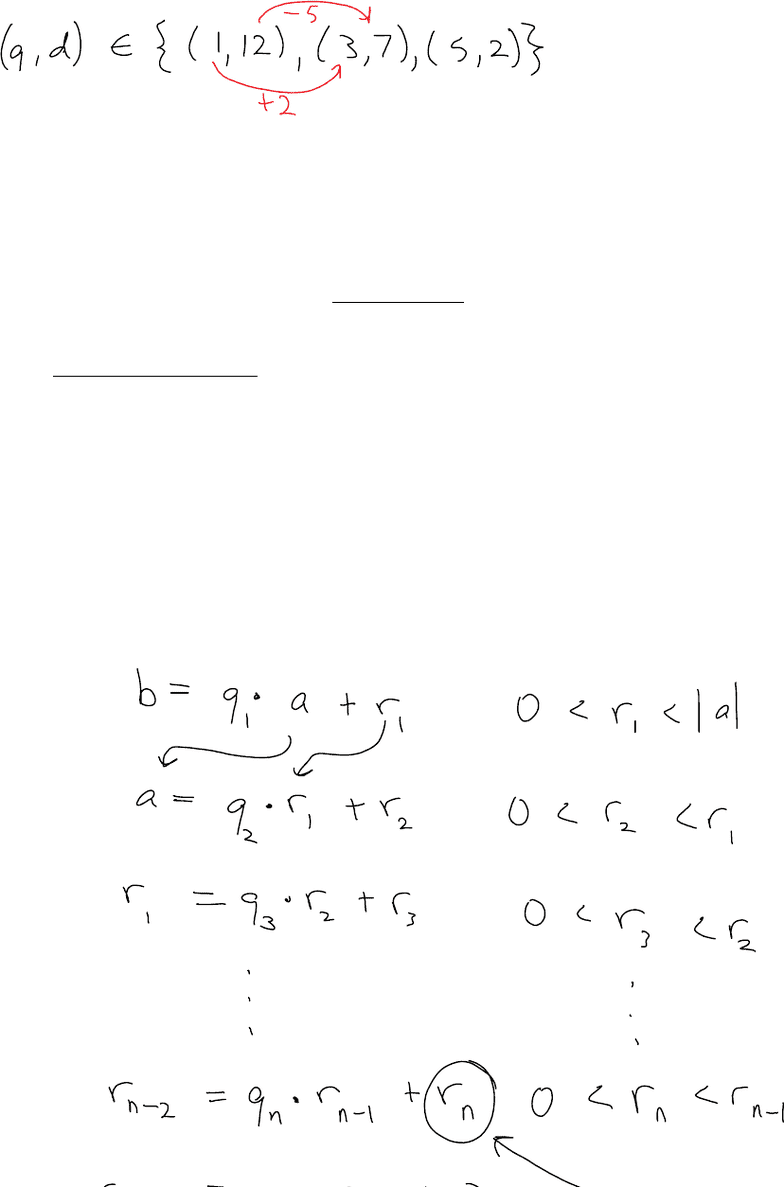# MACM 101 Lecture Notes - Lecture 12: Euclidean Algorithm, Mathematical Induction

18 views3 pages
School
Department
Course
ProfessorCameron has a lot of quarters and dimes with which to make change. How many different ways can he make change for
(a) \$1.39
(b) \$1.45
Solution
(a) since 5 doesn't divide 139, its not possible for Cameron to make change for \$1.39. (5|10 and 5|25 => 5|(10x + 25y)
(b)
First, try finding a single solution. Maybe use the most quarters: 5 quarters, 2 dimes. Now, 2 quarters = 5
dimes, so we can obtain 2 other solutions by swapping dimes for quarters.
Hence, 3 solutions are possible
For a, b, d integers, if d|a and d|b then d is a common divisor.
And if d satisfies:
whenever c|a and c|b then c|d
Then d is a greatest common divisor
If a, b are small, it is a fairly quick to find gcd(a,b) by trial and error/factor terms
But what if a, b is large
Problem: find gcd(481,1053)
The Euclidean Algorithm
Let a, b be intergers, a =/= 0. The gcd(a,b) equals the last nonzero remainder, rn, in the list of equations
obtained from the Division Algorithm
Lecture 12
February 1, 2016
10:24 AM
Lecture Notes Page 1
Unlock document

This preview shows page 1 of the document.
Unlock all 3 pages and 3 million more documents.

Already have an account? Log in

# Get access

Grade+
\$10 USD/m
Billed \$120 USD annually
Homework Help
Class Notes
Textbook Notes
40 Verified Answers
Study Guides
1 Booster Class
Class+
\$8 USD/m
Billed \$96 USD annually
Homework Help
Class Notes
Textbook Notes
30 Verified Answers
Study Guides
1 Booster Class